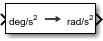Angular Acceleration Conversion

Convert from angular acceleration units to desired angular acceleration units

• Library:
• Aerospace Blockset / Utilities / Unit Conversions

•Description

The Angular Acceleration Conversion block computes the conversion factor from specified input angular acceleration units to specified output angular acceleration units and applies the conversion factor to the input signal.

The Angular Acceleration Conversion block port labels change based on the input and output units selected from the Initial unit and the Final unit lists.

Ports

Input

expand all

Angle, specified as a scalar, in initial acceleration units.

Dependencies

The input port label depends on the Initial unit setting.

Data Types: double

Output

expand all

Angle, returned as a scalar, in final acceleration units.

Dependencies

The output port label depends on the Final unit setting.

Data Types: double

Parameters

expand all

Specifies the input units, specified as:

 deg/s2 Degrees per second squared rad/s2 Radians per second squared rpm/s Revolutions per minute per second

Dependencies

The input port label depends on the Initial unit setting.

Programmatic Use

 Block Parameter: IU Type: character vector Values: 'deg/s^2' | 'rad/s^2' | 'rpm/s' Default: 'deg/s^2'

Output units, specified as:

 deg/s2 Degrees per second squared rad/s2 Radians per second squared rpm/s Revolutions per minute per second

Dependencies

The output port label depends on the Final unit setting.

Programmatic Use

 Block Parameter: OU Type: character vector Values: 'deg/s^2' | 'rad/s^2' | 'rpm/s' Default: 'rad/s^2'

Extended Capabilities

C/C++ Code GenerationGenerate C and C++ code using Simulink® Coder™.

Introduced before R2006a

Aerospace Blockset DocumentationGet trial now